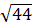### Sample Problem

A wooden board that is 12 feet long is leaning against a wall perpendicular to the ground. The point at which the wooden board touches the wall is 10 feet above the ground. How far away is the base of the board from the bottom of the wall? Round your answer to the nearest tenth.

feet

#### Solution

In this situation, the board represents the hypotenuse of a right triangle, and the height and base are the other two legs of a triangle. Using the Pythagorean Theorem, we have

a2 + 102 = 122

a2 = 44

a =a ≈ 6.6